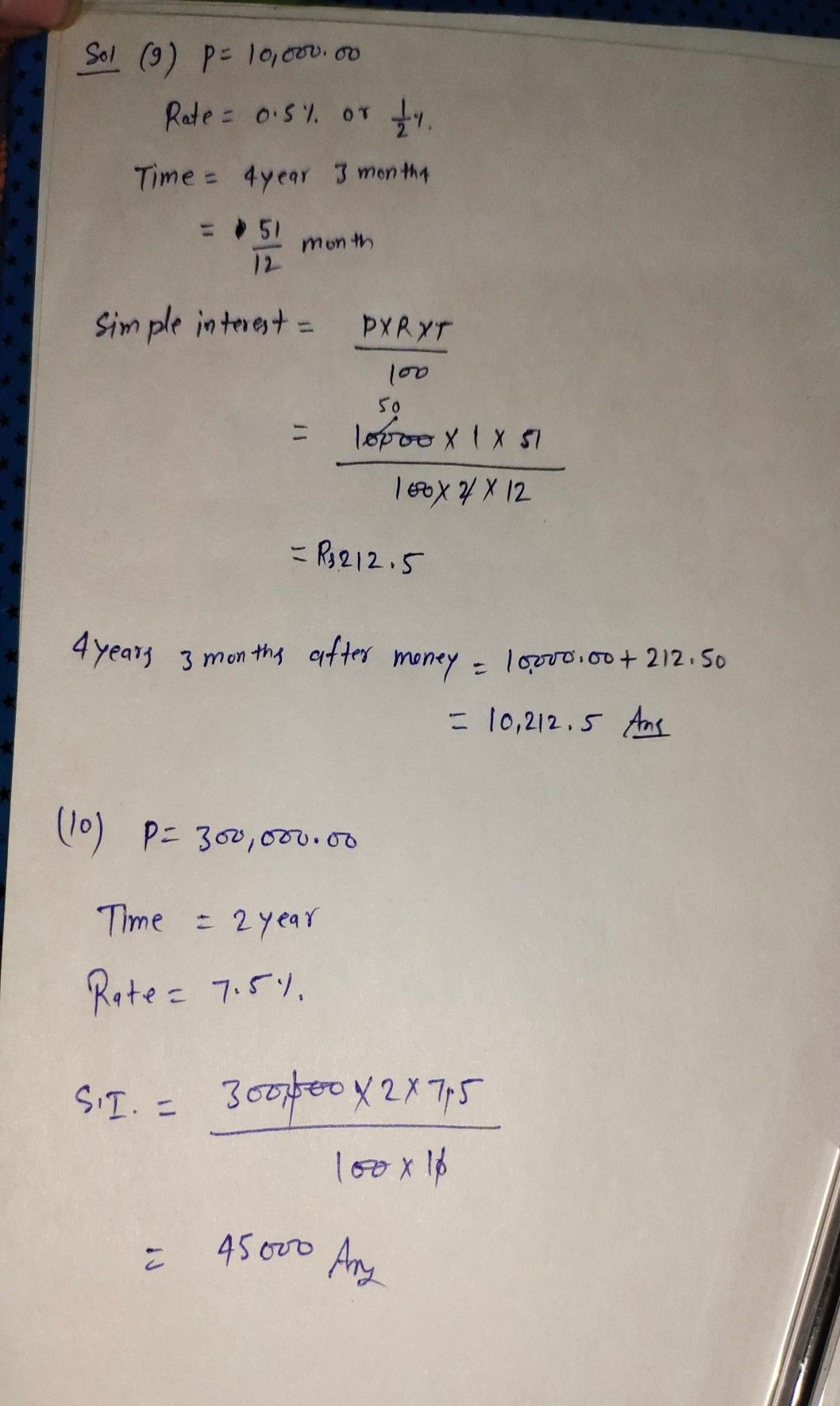Symbol
Problem$9.$ How much money will you have after $4$ years and $3$ months if you deposited $P10,000.00$ in a bank that pays $0.5%$ simple interest? $10$ How much should you invest at the simple interest is $7.5%$ in order to have $P300,000.00ln2$ years,? Po $c$
10th-13th grade
Other
Search count: 111
SolutionQanda teacher - shraddha